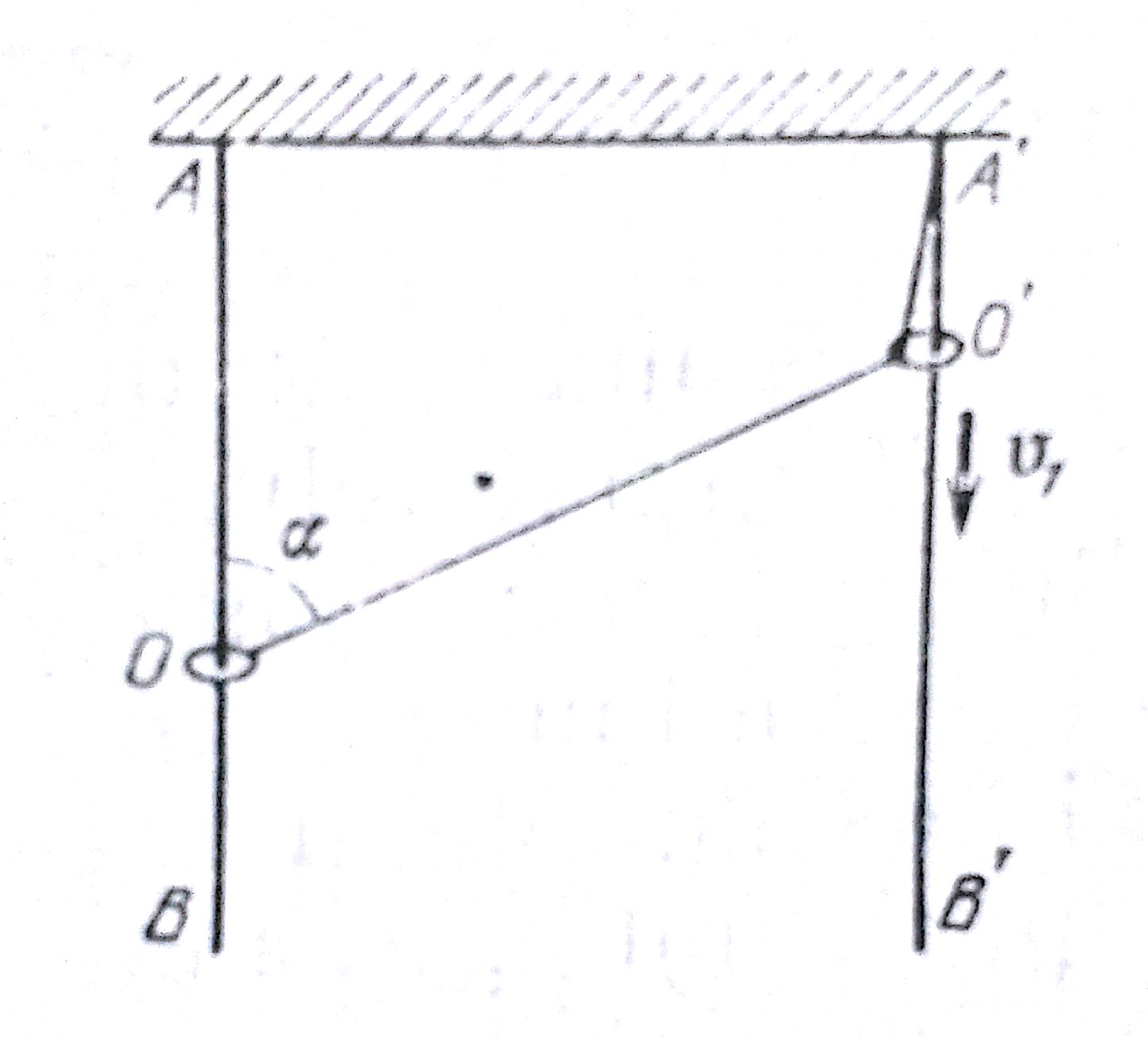# Ring-RaceThis question would amplify your skills in Kinematics.

Two rings O and O' are put two vertical stationary rods AB and A'B'. An inextensible thread is fixed at point A' and on the ring O and is passed through the ring O'. Assuming O' moves downwards constantly with $v_{1}$ , determine velocity $v_{2}$ if └AOO' is α. If the answer can be expressed as $v_{1}$a($sin^c(\frac{α}{b}$))/cosα. Find a+b+c

Also try : Optics or ClassicalMechanics

×

Problem Loading...

Note Loading...

Set Loading...